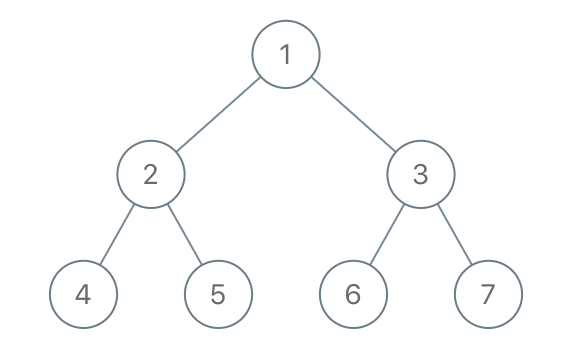GeetCode Hub

Given the root of a binary tree, each node in the tree has a distinct value.

After deleting all nodes with a value in to_delete, we are left with a forest (a disjoint union of trees).

Return the roots of the trees in the remaining forest. You may return the result in any order.

Example 1:Input: root = [1,2,3,4,5,6,7], to_delete = [3,5]
Output: [[1,2,null,4],,]

Example 2:

Input: root = [1,2,4,null,3], to_delete = 
Output: [[1,2,4]]

Constraints:

• The number of nodes in the given tree is at most 1000.
• Each node has a distinct value between 1 and 1000.
• to_delete.length <= 1000
• to_delete contains distinct values between 1 and 1000.

/** * Definition for a binary tree node. * public class TreeNode { * int val; * TreeNode left; * TreeNode right; * TreeNode() {} * TreeNode(int val) { this.val = val; } * TreeNode(int val, TreeNode left, TreeNode right) { * this.val = val; * this.left = left; * this.right = right; * } * } */ class Solution { public List<TreeNode> delNodes(TreeNode root, int[] to_delete) { } }